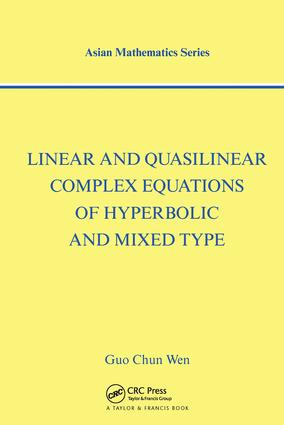# Linear and Quasilinear Complex Equations of Hyperbolic and Mixed Types

## 1st Edition

CRC Press

272 pages

##### Purchasing Options:\$ = USD
Hardback: 9780415269711
pub: 2002-08-22
Currently out of stock
\$145.00
x
eBook (VitalSource) : 9780429217593
pub: 2002-08-22
from \$28.98

FREE Standard Shipping!

### Description

This volume deals with first and second order complex equations of hyperbolic and mixed types. Various general boundary value problems for linear and quasilinear complex equations are investigated in detail. To obtain results for complex equations of mixed types, some discontinuous boundary value problems for elliptic complex equations are discussed. Mixed complex equations are included in the quasilinear case, and the text considers both boundary value conditions in the general oblique derivative case and multiply connected domains. Complex analytical methods are used to investigate various problems as well. In particular, hyperbolic numbers and hyperbolic complex functions are introduced to handle hyperbolic complex equations. Researchers and graduate students in mathematical analysis will find this text indispensable.

### Table of Contents

This volume deals with first- and second-order complex equations of hyperbolic and mixed types. The authors investigate in detail general boundary value problems for linear and quasilinear complex equations and present some discontinuous boundary value problems for elliptic complex equations. Mixed complex equations are included in the quasilinear case, and the text considers both boundary value conditions in the general oblique derivative case and multiply-connected domains. The authors also use complex analytical methods to investigate various problems. In particular, they introduce hyperbolic numbers and hyperbolic complex functions to handle hyperbolic complex equations.

### Subject Categories

##### BISAC Subject Codes/Headings:
MAT007000
MATHEMATICS / Differential Equations
MAT037000
MATHEMATICS / Functional Analysis